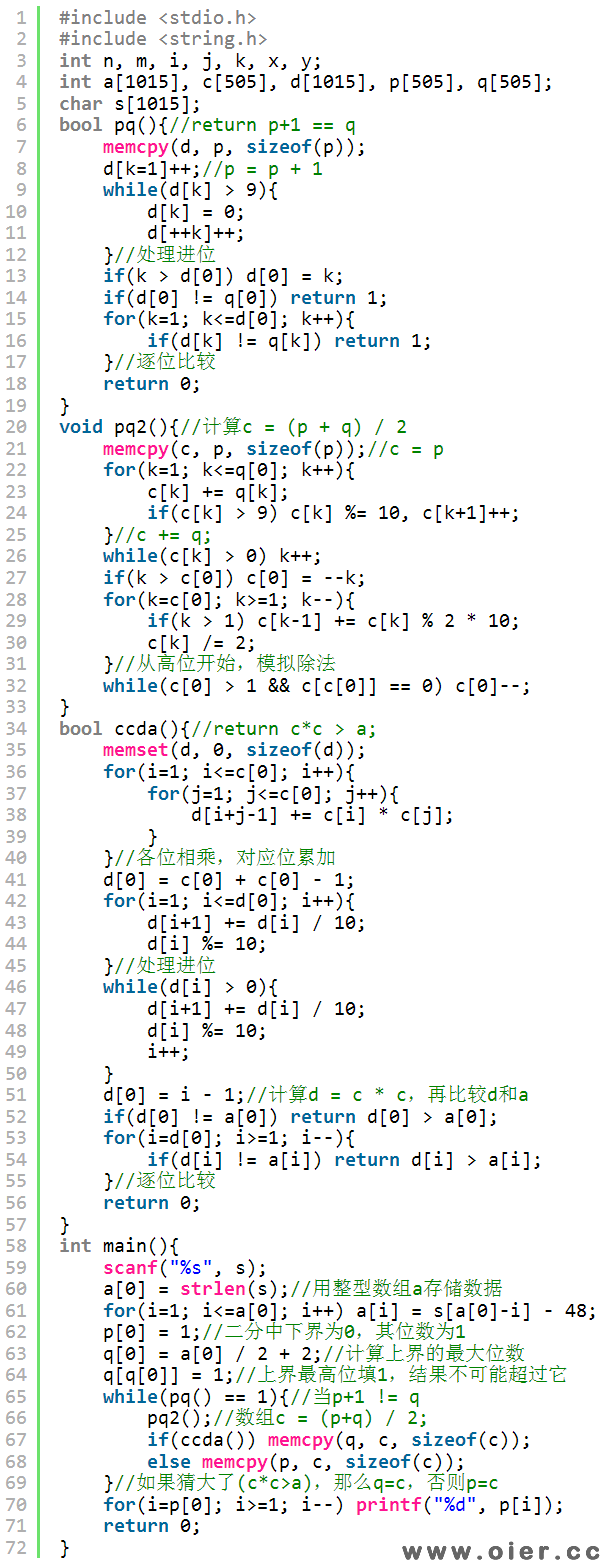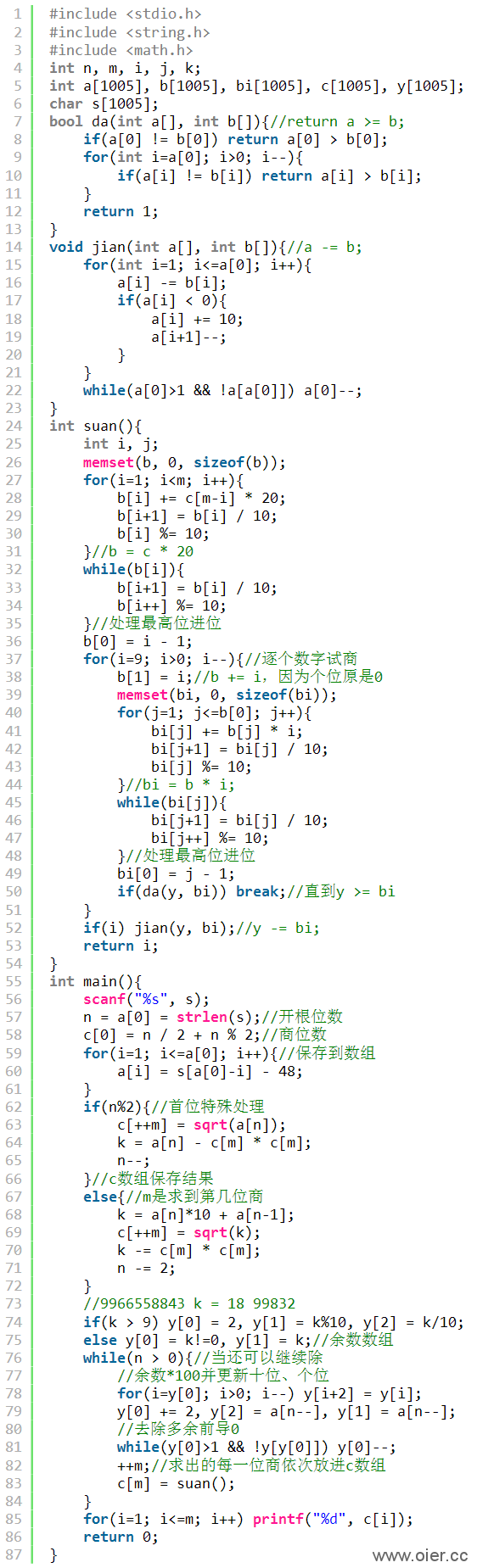CodeVS3119高精度练习之大整数开根
2060+

17

4

### 数据范围及提示 Data Size & Hint

n为不超过1000位的正整数 满足 1<=n <10^1000

# 解题思路

1、二分答案：结果约500位，二分答案只需要不到1670次，每次只需计算答案的平方（复杂度500^2），再与原数据进行比较。

2、模拟开方，复杂度O(n^2)。首先要会手算开方，不然如何模拟？

# 程序实现# 手算开根上图中，364是怎么来的？(9*20+2) * 2 = 437。因为(x+y)2 = x2 + 2xy + y2 = x2 + y * (2x+y)，所以922 = (90+2)2 = 902 + 2 * (2*90+2) = 902 + (9*20+2) * 2，因为902已经加过了，所以不需要再加。同样地，7376 = ( 92  * 20 +  4  ) *  4  。 更多 document.getElementById("bdshell_js").src = "http://bdimg.share.baidu.com/static/js/shell_v2.js?cdnversion=" + new Date().getHours();